### 1D Model of a Crystal Derivation *

We are working with the periodic potentialOur states have positive energy. This potential has the symmetry that a translation by the lattice spacingleaves the problem unchanged. The probability distributions must therefore have this symmetry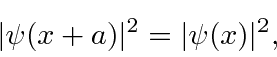which means that the wave function differs by a phase at most.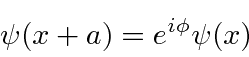The general solution in the regionisNow lets look at the boundary conditions at. Continuity of the wave function gives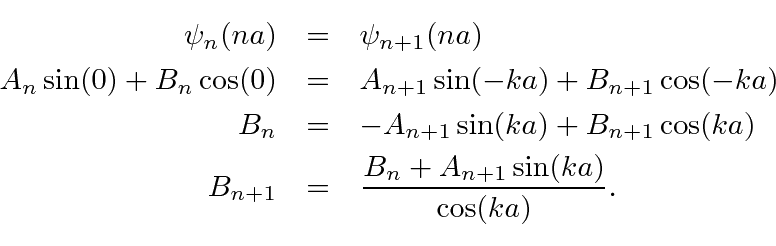The discontinuity in the first derivative isSubstituting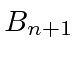from the first equationPlugging this equation for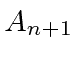back into the equation above forwe getWe now have two pairs of equations for the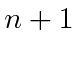coefficients in terms of thecoefficients.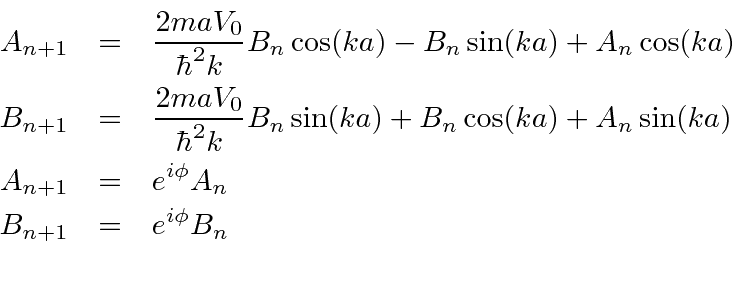Using the second pair of equations to eliminate thecoefficients, we have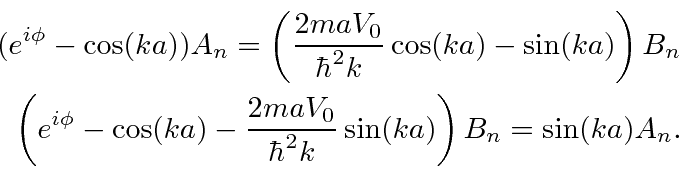Now we can eliminate all the coefficients.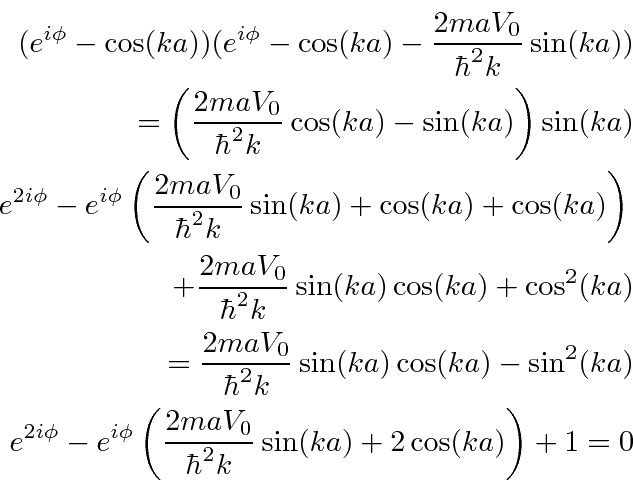Multiply by.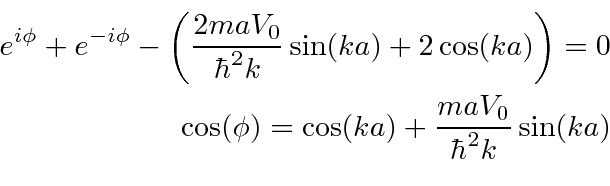This relation puts constraints on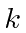, like the constraints that give us quantized energies for bound states. Since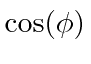can only take on values between -1 and 1, there are allowed bands ofand gaps between those bands.

Jim Branson 2013-04-22